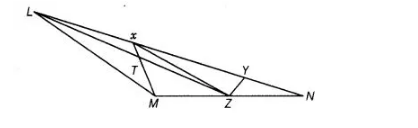# X and Y are points on the side L N of`
Question:

$X$ and $Y$ are points on the side $L N$ of the triangle $L M N$ such that $L X=X Y=Y N$. Through $X$, a line is drawn parallel to $L M$ to meet $M N$ at $Z$ (see figure). Prove that ar $(\Delta L Z Y)=\operatorname{ar}(M Z Y X)$.Thinking Process

Use the property that the triangles on the same base and between the same two parallel lines are equal in area. Further prove the required result.

Solution:

Given X and Y are points on the side LN such that

LX = XY = YN and XZ || LM To prove  ar (ΔLZY) = ar (MZYX)

Proof Since, ΔXMZ and ΔXLZ are on the same base XZ and between the same parallel lines LM and XZ.

Then, ar (ΔXMZ) = ar (ΔXLZ) …(i)

On adding ar (ΔXYZ) both sides of Eq. (i), we get

ar (ΔXMZ) + ar (ΔXXZ) = ar (ΔXLZ) + ar (ΔXYZ)

=> ar (MZYX) = ar (ΔLZY) Hence proved.